# Session 1 An Introduction to Valuation Aswath Damodaran

• Slides: 10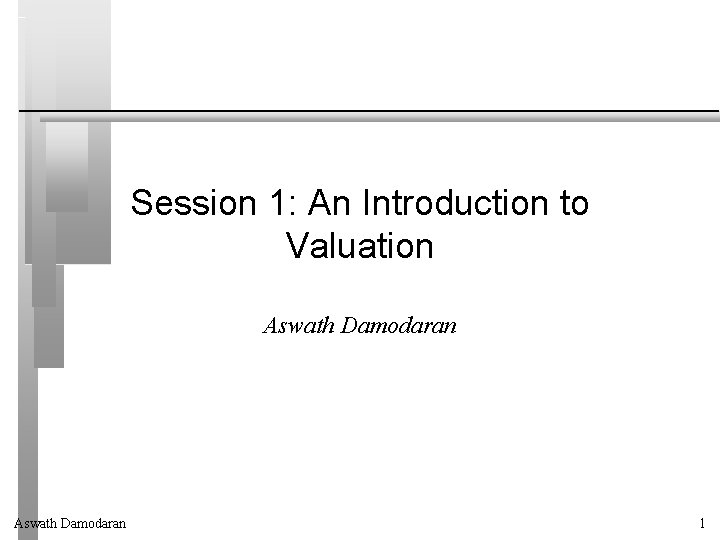Session 1: An Introduction to Valuation Aswath Damodaran 1Some Initial Thoughts " One hundred thousand lemmings cannot be wrong" We thought we were in the top of the eighth inning, when we were in the bottom of the ninth. . Stanley Druckenmiller Aswath Damodaran Graffiti 2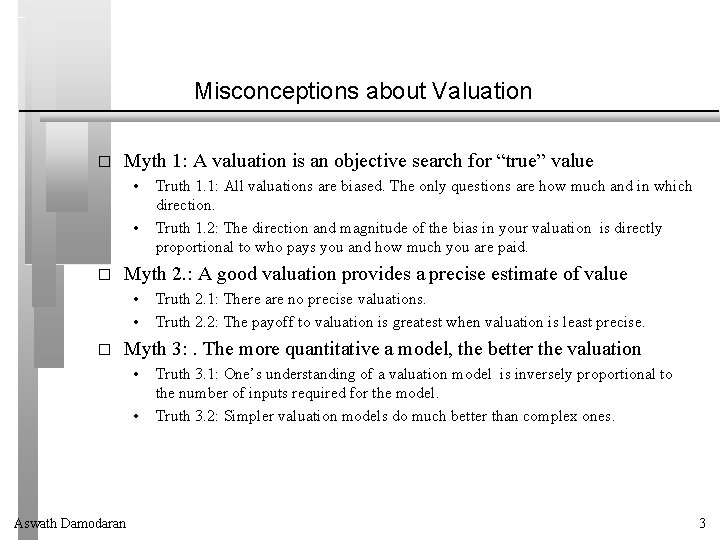Misconceptions about Valuation � Myth 1: A valuation is an objective search for “true” value • • � Myth 2. : A good valuation provides a precise estimate of value • • � Truth 1. 1: All valuations are biased. The only questions are how much and in which direction. Truth 1. 2: The direction and magnitude of the bias in your valuation is directly proportional to who pays you and how much you are paid. Truth 2. 1: There are no precise valuations. Truth 2. 2: The payoff to valuation is greatest when valuation is least precise. Myth 3: . The more quantitative a model, the better the valuation • • Aswath Damodaran Truth 3. 1: One’s understanding of a valuation model is inversely proportional to the number of inputs required for the model. Truth 3. 2: Simpler valuation models do much better than complex ones. 3Approaches to Valuation � � � Intrinsic valuation, relates the value of an asset to its intrinsic characteristics: its capacity to generate cash flows and the risk in the cash flows. In it’s most common form, intrinsic value is computed with a discounted cash flow valuation, with the value of an asset being the present value of expected future cashflows on that asset. Relative valuation, estimates the value of an asset by looking at the pricing of 'comparable' assets relative to a common variable like earnings, cashflows, book value or sales. Contingent claim valuation, uses option pricing models to measure the value of assets that share option characteristics. Aswath Damodaran 4Basis for all valuation approaches � The use of valuation models in investment decisions (i. e. , in decisions on which assets are under valued and which are over valued) are based upon • • � a perception that markets are inefficient and make mistakes in assessing value an assumption about how and when these inefficiencies will get corrected In an efficient market, the market price is the best estimate of value. The purpose of any valuation model is then the justification of this value. Aswath Damodaran 5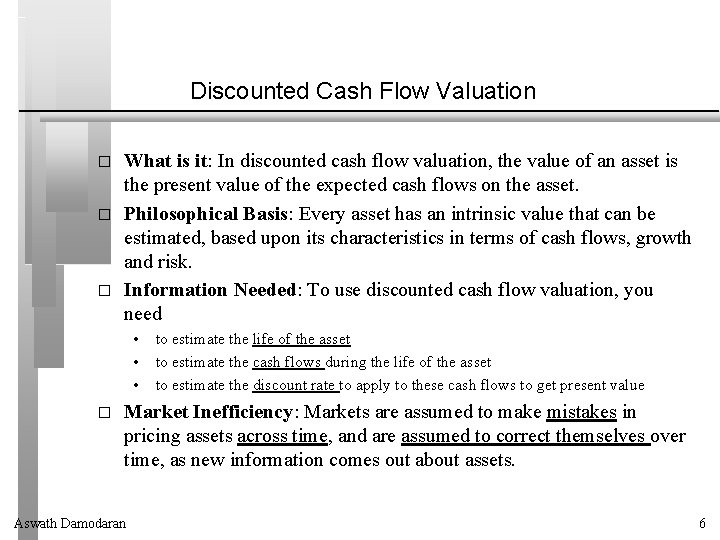Discounted Cash Flow Valuation � � � What is it: In discounted cash flow valuation, the value of an asset is the present value of the expected cash flows on the asset. Philosophical Basis: Every asset has an intrinsic value that can be estimated, based upon its characteristics in terms of cash flows, growth and risk. Information Needed: To use discounted cash flow valuation, you need • • • � to estimate the life of the asset to estimate the cash flows during the life of the asset to estimate the discount rate to apply to these cash flows to get present value Market Inefficiency: Markets are assumed to make mistakes in pricing assets across time, and are assumed to correct themselves over time, as new information comes out about assets. Aswath Damodaran 6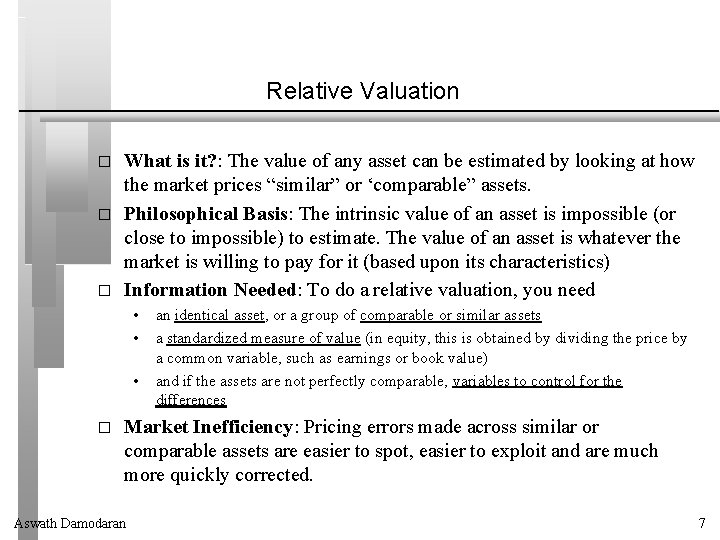Relative Valuation � � � What is it? : The value of any asset can be estimated by looking at how the market prices “similar” or ‘comparable” assets. Philosophical Basis: The intrinsic value of an asset is impossible (or close to impossible) to estimate. The value of an asset is whatever the market is willing to pay for it (based upon its characteristics) Information Needed: To do a relative valuation, you need • • • � an identical asset, or a group of comparable or similar assets a standardized measure of value (in equity, this is obtained by dividing the price by a common variable, such as earnings or book value) and if the assets are not perfectly comparable, variables to control for the differences Market Inefficiency: Pricing errors made across similar or comparable assets are easier to spot, easier to exploit and are much more quickly corrected. Aswath Damodaran 7Contingent Claim (Option) Valuation � � � What is it: In contingent claim valuation, you value an asset with cash flows contingent on an event happening as options. Philosophical Basis: When you buy an option-like asset, you change your risk tradeoff – you have limited downside risk and almost unlimited upside risk. Thus, risk becomes your ally. Information Needed: To use contingent claim valuation, you need • • • � define the underlying asset on which you have the option a conventional value for your asset, using discounted cash flow valuation the contingency that will trigger the cash flow on the option Market Inefficiency: Investors who ignore the optionality in optionlike assets will misprice them. Aswath Damodaran 8Indirect Examples of Options � � Equity in a deeply troubled firm - a firm with negative earnings and high leverage - can be viewed as an option to liquidate that is held by the stockholders of the firm. Viewed as such, it is a call option on the assets of the firm. The reserves owned by natural resource firms can be viewed as call options on the underlying resource, since the firm can decide whether and how much of the resource to extract from the reserve, The patent owned by a firm or an exclusive license issued to a firm can be viewed as an option on the underlying product (project). The firm owns this option for the duration of the patent. The rights possessed by a firm to expand an existing investment into new markets or new products. Aswath Damodaran 9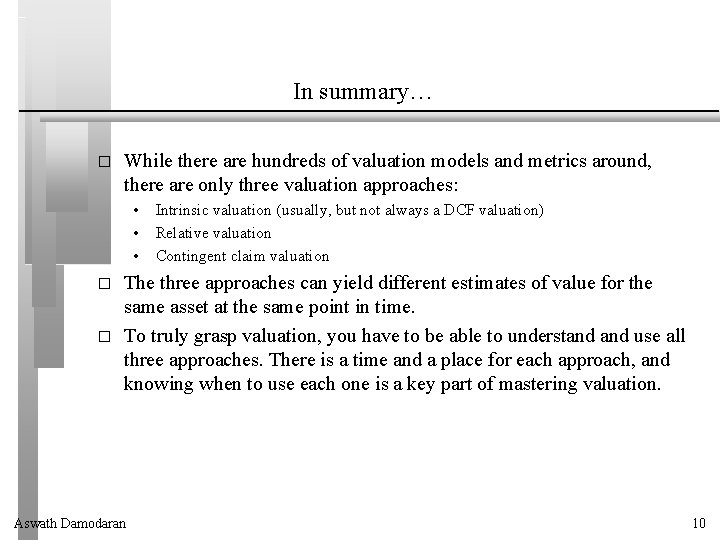In summary… � While there are hundreds of valuation models and metrics around, there are only three valuation approaches: • • • � � Intrinsic valuation (usually, but not always a DCF valuation) Relative valuation Contingent claim valuation The three approaches can yield different estimates of value for the same asset at the same point in time. To truly grasp valuation, you have to be able to understand use all three approaches. There is a time and a place for each approach, and knowing when to use each one is a key part of mastering valuation. Aswath Damodaran 10